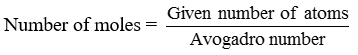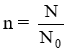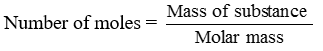Ex﻿

3. Atoms & Molecules

Mole Concept

Atoms and molecules are so small in size that they cannot be counted individually. The chemists use the unit mole for counting atoms, molecules or ions. It is represented by n. A mole represents 6.022 × 1023 particles.
Example. 1 mole of atoms = 6.022 × 1023 atoms.
1 mole of molecules = 6.022 × 1023 molecules The number of particles present in 1 mole of any substance is fixed i.e. 6.022 × 1023.
This number is called Avogadro constant or Avogadro number. It is represented by No. 1 mole of atoms = 6.022 × 1023 atoms = Gram atomic mass or Molar mass of elementNo. of moles = n
Given mass = m
Molar mass = M
Given number of particles = N
Avogadro number of particles = N0
These relations can be interchanged as
Mass of element, m = n × M
or No. of particles of element, N = n × N0
Similarly,
1 Mole of molecules = 6.022 × 1023 molecules
= Gram molecular mass of Molar massor m = n × M and N = n × N0

If you want to give information about online courses to other students, then share it with more and more on Facebook, Twitter, Google Plus. The more the shares will be, the more students will benefit. The share buttons are given below for your convenience.
×

NTSE Chemistry (Class X)

• Metals & Non Metals
• Elementary Idea of Bonding
• Chemical Reactions
• Acid Bases & Salts
• Compounds of Common Use
• Carbon and Its Compound
• Periodic Classification of Elements

NTSE Chemistry (Class IX)

• Matter in our Surrounding
• Is Matter Around us Pure
• Atoms & Molecules
• Structure of Atoms

SHOW CHAPTERS